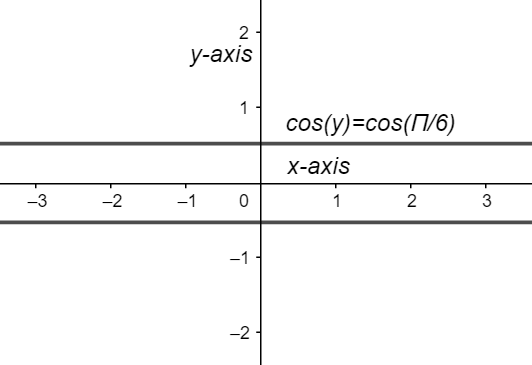Courses
Courses for Kids
Free study material
Offline Centres
MoreLast updated date: 08th Dec 2023
Total views: 281.7k
Views today: 3.81k

# Find the value of ${{\cos }^{-1}}\left( \cos \dfrac{13\pi }{6} \right)$?Verified
281.7k+ views
Hint: Equate the trigonometric function to a variable. And then find the ranges of the $\cos$ functions and solve for them. Find the value that satisfies the condition. Substitute the angle of $\pi$ and evaluate it.

Complete step-by-step solution:
Let us learn about inverse trigonometric functions. Inverse trigonometric functions are also known as anti trigonometric functions, arcus functions, and cyclometric functions. These inverse trigonometric functions formulas enable us to find out any angles with any of the trigonometry ratios. The inverse trigonometric function does exactly function the opposite way of the normal trigonometric functions. The -1 in the exponent of the trigonometric is not the exponent but it is the symbol for inverse function. The range of ${{\cos }^{-1}}$ function is $\left( 0,\pi \right)$.
Now let us find the value of the given trigonometric function ${{\cos }^{-1}}\left( \cos \dfrac{13\pi }{6} \right)$
Let $y={{\cos }^{-1}}\left( \cos \dfrac{13\pi }{6} \right)$
$\dfrac{13\pi }{6}=\dfrac{13\times 180}{6}={{390}^{\circ }}$
Multiply with $\cos$ on both sides, we get
$\Rightarrow \cos y=\cos \dfrac{13\pi }{6}$
On substituting the value of $\dfrac{\pi }{6}$, we get, i.e. $\dfrac{13\pi }{6}=\dfrac{13\times 180}{6}=13\times 30={{390}^{\circ }}$
$\Rightarrow \cos y=\cos \left( {{390}^{\circ }} \right)$
As we know that the range of principal values of $\cos$ is $\left(0, \pi \right)$.
$\therefore y={{390}^{\circ }}$ is not possible.
Now,
\begin{align} & \Rightarrow \cos y=\cos \left( {{390}^{\circ }} \right) \\ & \Rightarrow \cos y=\cos \left( {{360}^{\circ }}+{{30}^{\circ }} \right) \\ & \Rightarrow \cos y=\cos {{30}^{\circ }} \\ & \Rightarrow \cos y=\cos \left( {{30}^{\circ }}\times \frac{\pi }{180} \right) \\ & \Rightarrow \cos y=\cos \left( \frac{\pi }{6} \right) \\ & \therefore y=\frac{\pi }{6} \\ \end{align}
This is in the range of principle values of ${{\cos }^{-1}}$ is $\left( 0,\pi \right)$.
$\therefore$ ${{\cos }^{-1}}\left( \cos \dfrac{13\pi }{6} \right)$=$y=\dfrac{\pi }{6}$.
The graph of the given function isNote: Here is the list of properties of inverse trigonometric functions.
\begin{align} & \sin \left( {{\sin }^{-}}^{1}x \right)\text{ }=\text{ }x,\text{ }if\text{ }-1\text{ }\le \text{ }x\text{ }\le \text{ }1 \\ & \cos \left( {{\cos }^{-}}^{1}x \right)\text{ }=\text{ }x,\text{ }if\text{ }-1\text{ }\le \text{ }x\text{ }\le \text{ }1 \\ & \tan \left( {{\tan }^{-}}^{1}x \right)\text{ }=\text{ }x,\text{ }if\text{ }-\infty \text{ }\le \text{ }x\text{ }\le \infty \\ & \cot \left( {{\cot }^{-}}^{1}x \right)\text{ }=\text{ }x,\text{ }if\text{ }-\infty \le \text{ }x\text{ }\le \infty \\ & \sec \left( {{\sec }^{-}}^{1}x \right)\text{ }=\text{ }x,\text{ }if\text{ }-\infty \text{ }\le \text{ }x\text{ }\le \text{ }-1\text{ }or\text{ }1\text{ }\le \text{ }x\text{ }\le \text{ }\infty \\ & \csc \left( {{\csc }^{-}}^{1}x \right)\text{ }=\text{ }x,\text{ }if\text{ }-\infty \text{ }\le \text{ }x\text{ }\le \text{ }-1\text{ }or\text{ }1\text{ }\le \text{ }x\text{ }\le \text{ }\infty \\ \end{align}
The inverse trigonometric relations are not functions because for any given input there exists more than one output. That is, for a given number there exists more than one angle whose sine, cosine, etc., is that number.